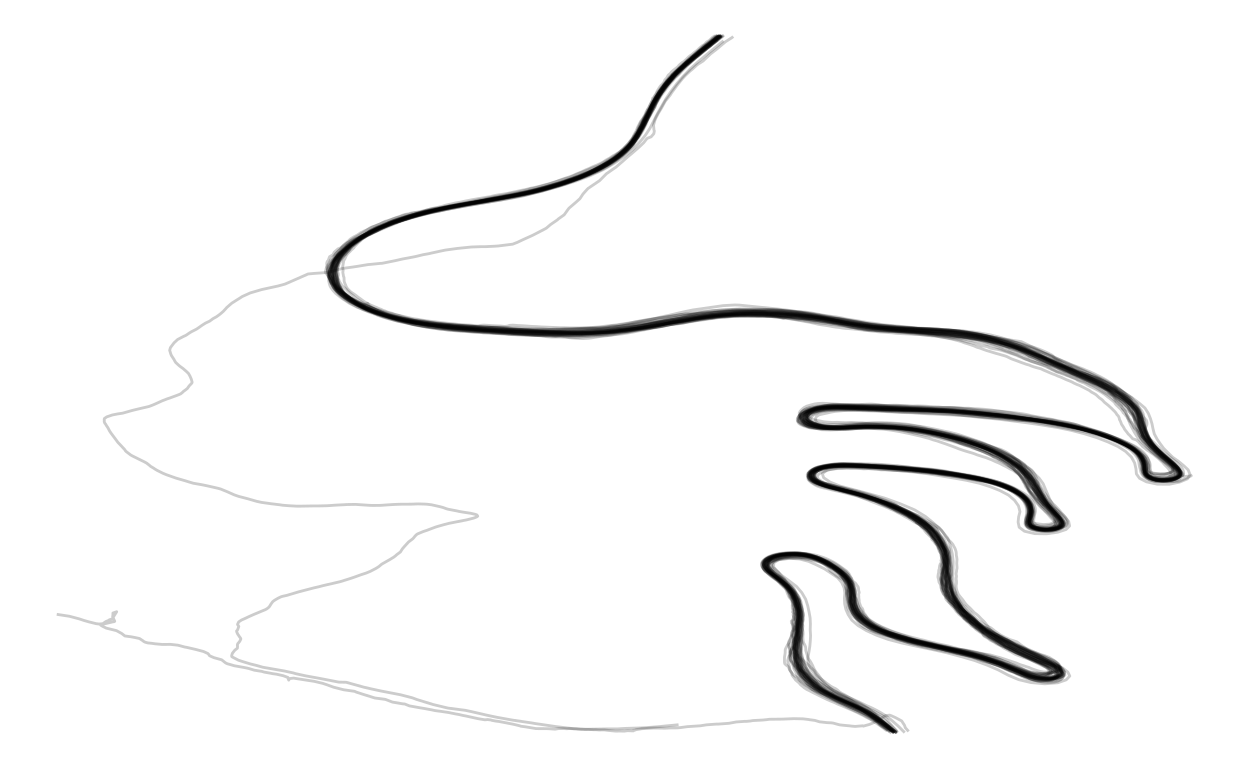# Mountain Race

Animation of my Strava efforts on one of my local climbs

Julian During
2022-03-30

# Idea

Every cyclist has a particular important climb. It might not be a big deal to anyone else, but any climb can be important!

My favorite local climb goes by the name of ‘Lochen’. It’s located outside of my local hometown Balingen in the southwest of Germany. It’s about 4.4 kilometers long with an average gradient of 6.9%.

This doesn’t sound like a hard climb. It might not even register as a regular big climb for most cyclists. But for me it’s one of the most iconic climbs.

In the following post, I will let different versions of me race against each other on my favorite local climb!

In order to reproduce the analysis, perform the following steps:

• Clone the repository
• Install the packages listed in the libraries.R file
• Run the target pipeline by executing targets::tar_make() command

# Data

The data originates from my personal Strava account. If you have a Strava account and want to query your data like I do here, you can have a look at one of my previous posts.

The data are a bunch of arrow files, that you can query via dpylr syntax thanks to the DuckDB package.

Deselect heartrate measurements and restrict the spatial data to a bounding box. Add information about the type and the start date of each activity.

poi <- function(df_act, paths_meas, target_file, act_type,
lng_min, lng_max, lat_min, lat_max) {
act_col_types <- schema(
moving = boolean(), velocity_smooth = double(),
grade_smooth = double(), distance = double(),
altitude = double(), heartrate = int32(), time = int32(),
lat = double(), lng = double(), cadence = int32(),
watts = int32(), id = string())

strava_db <- open_dataset(
paths_meas, format = "arrow", schema = act_col_types) |>
to_duckdb()

df_strava_poi <- strava_db |>
filter(
lng >= lng_min, lng <= lng_max, lat >= lat_min, lat <= lat_max) |>
select(-heartrate) |>
collect() |>
left_join(select(df_act, id, type, start_date), by = "id")
}

# A tibble: 40,439 × 13
moving velocity_smooth grade_smooth distance altitude  time   lat
<lgl>            <dbl>        <dbl>    <dbl>    <dbl> <int> <dbl>
1 TRUE               3            3.4   22882.     637.  3925  48.2
2 TRUE               3            1.7   22885.     637.  3926  48.2
3 TRUE               2.9          3.4   22887.     637   3927  48.2
4 TRUE               3            3.4   22890.     637   3928  48.2
5 TRUE               2.9          3.3   22893.     637.  3929  48.2
6 TRUE               3            3.3   22896.     637.  3930  48.2
7 TRUE               2.9          3.3   22899.     637.  3931  48.2
8 TRUE               3            5     22902.     637.  3932  48.2
9 TRUE               3            4.9   22905.     638.  3933  48.2
10 TRUE               3            6.7   22908.     638.  3934  48.2
# … with 40,429 more rows, and 6 more variables: lng <dbl>,
#   cadence <int>, watts <int>, id <chr>, type <chr>,
#   start_date <dttm>

Further preprocess the raw data. Keep only rows, where I was moving and turn the start date from datetime to date. Adjust the time column so that every activity starts at time 0.

lochen <- function(df_lochen_raw) {
df_lochen_raw |>
group_by(id) |>
mutate(
time_delta = time - lag(time),
start_date = as_date(start_date)) |>
replace_na(list(time_delta = 0)) |>
filter(moving) |>
mutate(time = cumsum(time_delta)) |>
select(-time_delta)
}

# A tibble: 40,365 × 13
# Groups:   id 
moving velocity_smooth grade_smooth distance altitude  time   lat
<lgl>            <dbl>        <dbl>    <dbl>    <dbl> <int> <dbl>
1 TRUE               3            3.4   22882.     637.     0  48.2
2 TRUE               3            1.7   22885.     637.     1  48.2
3 TRUE               2.9          3.4   22887.     637      2  48.2
4 TRUE               3            3.4   22890.     637      3  48.2
5 TRUE               2.9          3.3   22893.     637.     4  48.2
6 TRUE               3            3.3   22896.     637.     5  48.2
7 TRUE               2.9          3.3   22899.     637.     6  48.2
8 TRUE               3            5     22902.     637.     7  48.2
9 TRUE               3            4.9   22905.     638.     8  48.2
10 TRUE               3            6.7   22908.     638.     9  48.2
# … with 40,355 more rows, and 6 more variables: lng <dbl>,
#   cadence <int>, watts <int>, id <chr>, type <chr>,
#   start_date <date>

# Visualisation

Make a first static ggplot visualisation. Keep the plot rather minimal. Use ggplot2::theme_void as a general theme:

vis_lochen <- function(df_lochen) {
df_lochen |>
ggplot(
aes(x = lng, y = lat, group = id)) +
geom_path(alpha = 0.2) +
theme(
axis.ticks.x = element_blank(), legend.position = "bottom") +
labs(x = element_blank(), y = element_blank(), color = "Activity Year")
}As you can see there are lot of paths on one road. These are my bike rides on the ‘Lochen’ pass.

Some paths don’t seem to match. These are activities of another type in the same region as my bike rides. These activities don’t use the main road and stand out in the plot.

To further explore the data, make a first animated visualisation with the gganimate package:

vis_anim_lochen <- function(gg_lochen) {
gg_lochen +
transition_reveal(along = time)
}In this animated version of the plot, you can see that there are further problems in the data. Not all bike rides start at the bottom of the climb. You can guess which activities start at the top of the climb, by looking at the general speed of the animation. Determine these activities:

wrong_direction <- function(df_lochen) {
df_lat_lng_start <- df_lochen |>
group_by(id) |>
summarise(
start_lat = lat[time == min(time)], start_lng = lng[time == min(time)])

midway <- min(df_lochen$lat) + (max(df_lochen$lat) - min(df_lochen\$lat)) / 2

df_lat_lng_start |>
filter(start_lat < midway)
}


Filter the activities for bike rides. Exclude activities that start at the top of the climb. Repeat the above animated plot:

lochen_ride <- function(df_lochen, df_wrong_direction) {
df_lochen |>
filter(type == "Ride") |>
anti_join(df_wrong_direction, by = "id")
}